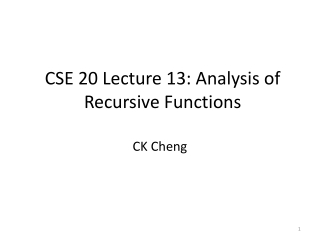DownloadDownload PresentationCSE 20 Lecture 13: Analysis of Recursive Functions

# CSE 20 Lecture 13: Analysis of Recursive Functions

Download Presentation## CSE 20 Lecture 13: Analysis of Recursive Functions

- - - - - - - - - - - - - - - - - - - - - - - - - - - E N D - - - - - - - - - - - - - - - - - - - - - - - - - - -
##### Presentation Transcript

1. 3. Analysis • 3.1 Introduction • 3.2 Homogeneous Linear Recursion

2. 3.1 Introduction Derive the bound of functions or recursions Estimate CPU time and memory allocation Eg. PageRank calculation Allocation of memory, CPU time, Resource optimization MRI imaging Real time? VLSI design Design automation flow to meet the deadline for tape out? Further Study Algorithm, Complexity

3. 3.1 Introduction • Derive the bound of functions or recursions • Estimate CPU time and memory allocation • Example on Fibonacci Sequence: Estimate fn. • Index: 0 1 2 3 4 5 6 7 8 9 • fn: 0 1 1 2 3 5 8 13 21 34

4. Example: Fibonacci Sequence

5. Example: Fibonacci Sequence, iClicker Suppose g0=0 and g1=2, gn=gn-1+gn-2what is gn • A. gn=fn • B. gn=2fn • C. gn=3fn • D. None of the above

6. 3.2 Homogeneous Linear Recursion • (1) Arithmetic Recursion a, a+d, a+2d, …, a+kd • (2) Geometric Recursion a, ar, ar2, …, ark • (3) Linear Recursion an= e1an-1+e2an-2+…+ekan-k+ f(n)

7. Linear Recursion and Homogeneous Linear Recursion • Linear Recursion: There are no powers or products of • Homogenous Linear Recursion: A linear recursion with f(n)=0.

8. Solving Linear Recursion • Input: Formula • and k initial values • Output: an as a function of n • 1. Set characteristic polynomial: • 2. Find the root of the characteristic polynomial (assuming ri are distinct) • 3. Express • 4. Determine ci from k initial values

9. Solving Linear Recursion Input: Formula and k initial values 1. Set characteristic polynomial: Rewrite the formula with n=k Replace ai with xi

10. Solving Linear Recursion Input: Formula and k initial values 2. Find the root of the polynomial Or,

11. Solving Linear Recursion Input: Formula and k initial values 3. Express (assuming that the roots are distinct.) 4. Determine ci from k initial values

12. Solving Linear Recursion Input: Formula and k initial values 3. Set (when the roots are not distinct.) where ri is a root of multiplicity wi

13. Example on Fibonacci sequence Input: initial values a0=0 and a1=1; and recursion formula an=an-1+an-2. Rewrite recursion: an-an-1-an-2=0. Or a2-a1-a0=0. 1. Characteristic polynomial: x2-x-1=0. 2. Roots of the polynomial: 3. Set: an=c1r1n+c2r2n.

14. Example on Fibonacci sequence Input: initial values a0=0 and a1=1; and recursion formula an=an-1+an-2. 4. Determine ci from k initial values a0=c1r10+c2r20 => c1+c2=0 a1=c1r11+c2r21 => c1r1+c2r2=1. Thus, we have an=c1r1n+c2r2n, where

15. Example 2

16. Example 2 (cont) Two initial values a0=c1+c2: c1+c2=1 a1=2c1+(-1)c2: 2c1-c2=1 Thus, we have c1=2/3, c2=1/3. Since an=c1r1n+c2r2n, we have an=2/3×2n+1/3×(-1)n,

17. Example 3